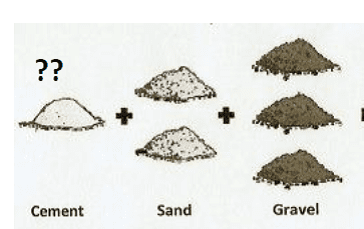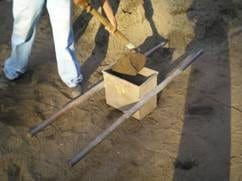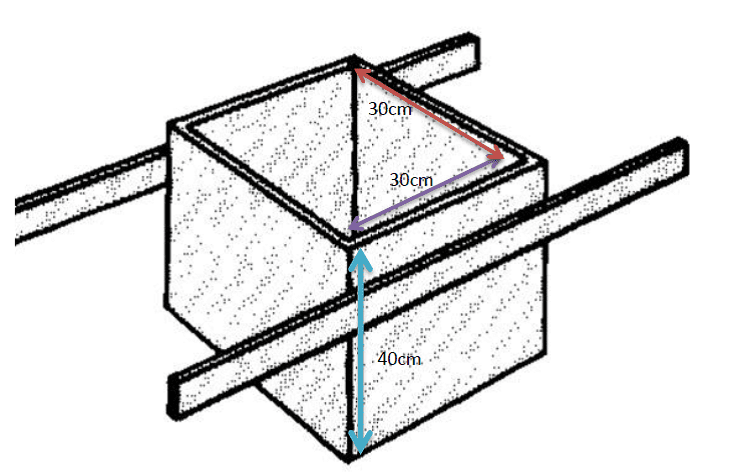# What is the procedure to calculate cement bags in 1 cubic meter of concrete?

To arrive at different quantities of concrete ingredients, the Dry loose bulk density of materials plays important role as concrete ingredients are batched loosely in volume proportion.## How to Determine Dry Loose Bulk Density of Materials

### a. Calculating Dry Loose Bulk Density of Cement

Cement being a factory made product and packaged in Bags weighing 50 kg each,its DLBD more or less fall around 1400 kg/cum.

Note: 1 cum = 1000 liters

For 50 kg (01 bag cement) the volume arrived is

1400 kg cement occupies Volume of 1 cum or 1000 liter (DLBD = 1400 kg/cum)

50kgs of cement will have volume, = 50/1400 = ~ 36 liter

Alternatively you can find the dry loose density as follows:

Have a 20 liter (V1) capacity containerTake its empty weight = W1

Fill loosely cement in the container to the full height, measure weight of container with cement = W2

Density= Mass/Volume = (W2 – W1) / (V1) = ‘x’ Kg/liter

DLBD of Cement = ‘x’ Kg/liter

For arriving at DLBD of Aggregates/Gravel, repeat the above procedure with coarse aggregate (stone chips) and fine aggregate (Sand).

Note: Ensure the aggregate are dry (sand if wet will be bulky, bulkiness depend on wet %), filled loosely not compacted (at site practically not feasible to compact concrete ingredients for each batch of concrete) and to the full height of the container.Measuring Coarse and Fine Aggregate at Site using Measuring Box

### B. Calculation of Number of Bags of Cement for 1 Cum Concrete

Considering Dry loose bulk density of following concrete ingredients as

Cement = 1400 kg/cum = 1.4 kg/ltr.

Fine Aggregate = 1600 kg/cum or 1.6 kg/ltr.(depends on source, gradation)

Coarse Aggregate = 1450 kg/cum = 1.45 kg/ltr. (Depend on source, type, shape, size)

Water = 1000 kg/cum = 1 kg/ltr.(Universal Constant)

Considering a concrete mix proportion (by volume)

1:2:4:: cement: fine aggregate : Coarse Aggregate

Cement required = 01 bag = 36 liters (36 cc) = 50 kg

For batching ease, the farma/measuring box, dimensions should be selected to match volume of a cement bag i.e. 36 liter or 36 cc (cubic centimeter), as the cement is added per bagBox size = 40cmx30cmx30cm = 36 cc (Cubic cm)

Fine Aggregate required = 02*36 = 72 ltr. = 72*1.6 = 115 kg

Coarse Aggregate required = 04*36 = 144 ltr.=144*1.45= 209 kg

Considering, water – cement ratio (w/c) of 0.55, water = 0.55*50 = 27.5 kg

The volume proportions (1:2:4) turns out to be

50 :115 : 209 :: 1: 2.3 : 4.18 by weight, Water= 27.5

Total weight of concrete ingredients = 401.5 say 400 kg

Density of concrete = 2400 kg/cum

01 batch (1:2:4) produces = 400/2400 = 0.167 cum of concrete

No. of bags required for 01 cum of concrete = 1/0.167 = 6 bags

From above, if the concrete mix is 1:2:4(by volume), to get a cubic meter of concrete we require

1. Cement = 6 bags = 300 kgs.
2. Fine Aggregate = 115/0.167 = 689 kgs = 15.2 cft
3. Coarse Aggregate = 209/0.167 = 1252 kgs = 30.5 cft
4. Water = 300/0.55 (w/c) = 165 kg/liter.

Likewise other concrete mix with varying proportions and materials with different physical properties, the individual quantities of different ingredients can be easily arrived.